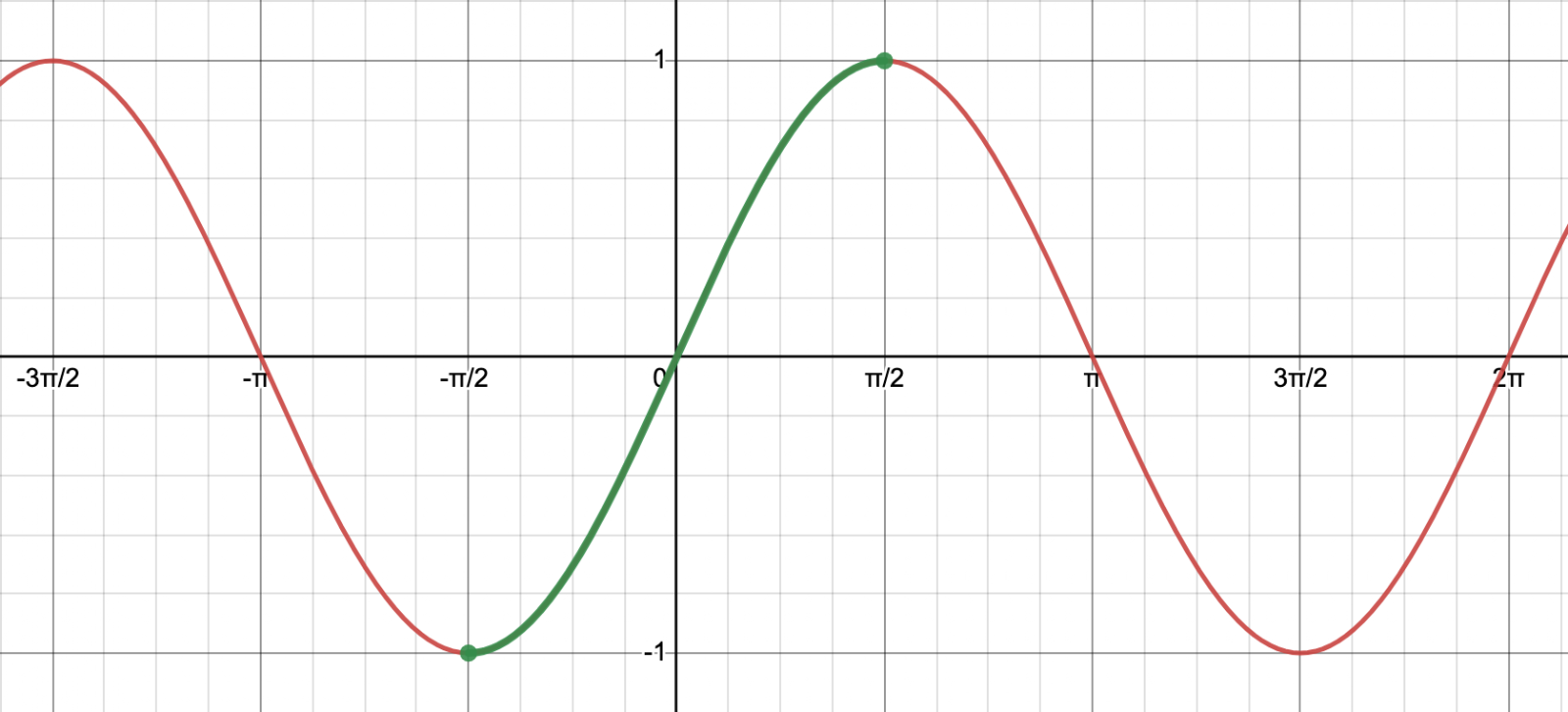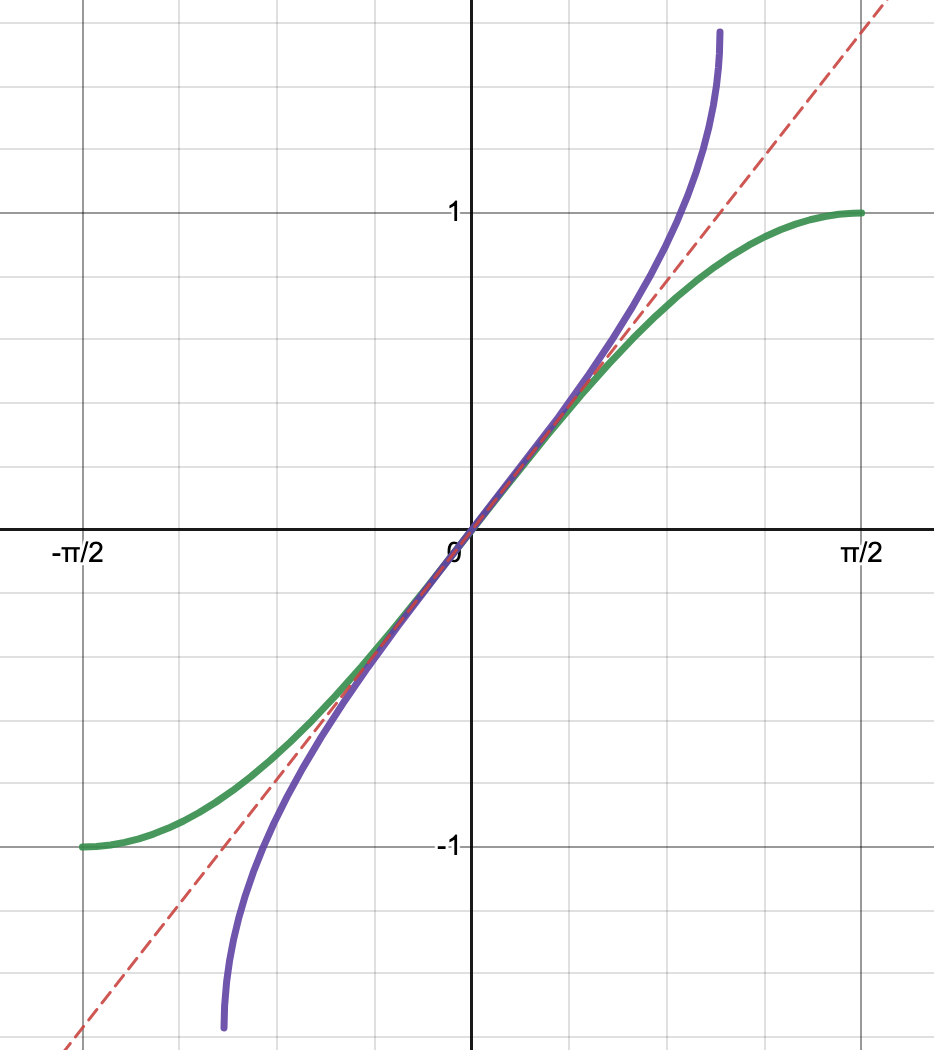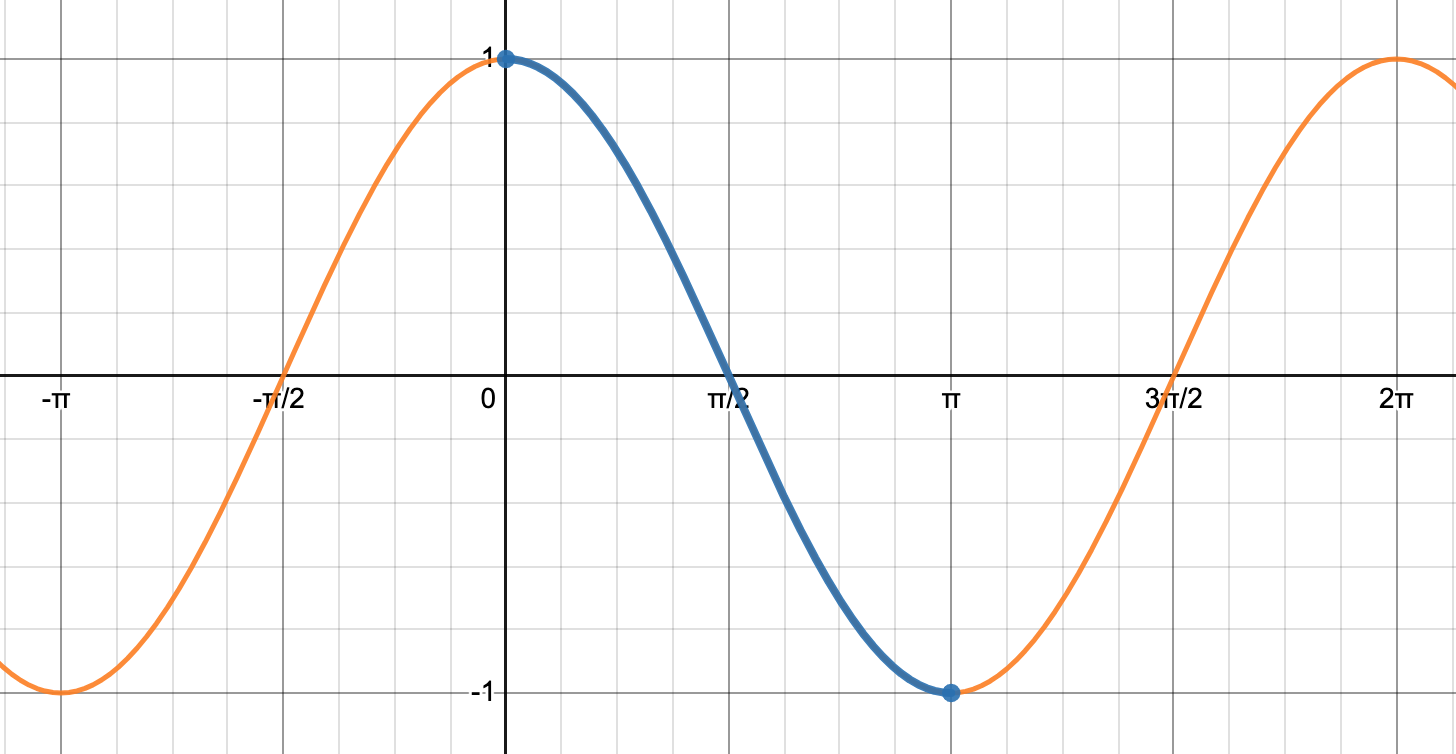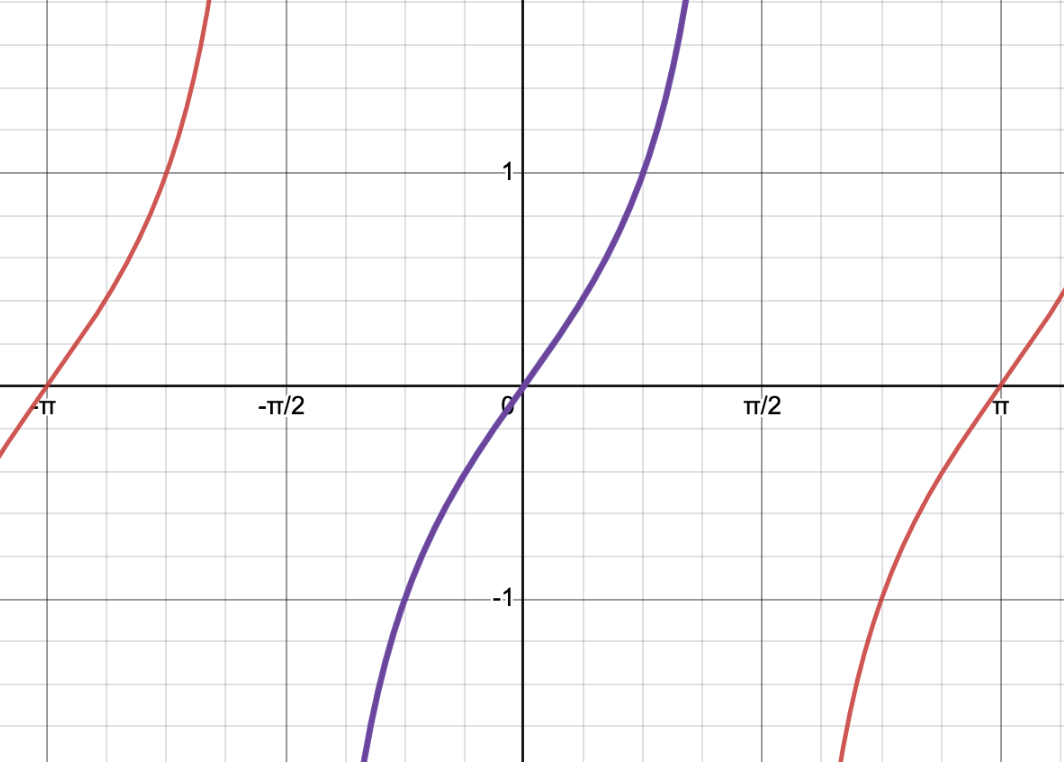# Inverse Trigonometric Functions (6 To Learn)

Recall that in order for a function to have an inverse function, it must be one-to-one or pass the Horizontal Line Test (HLT). None of the six trigonometric functions pass the Horizontal Line Test but we can restrict the domain of the original functions so that the functions will be one-to-one.

The general idea is to find the largest possible natural domain centered near the origin that will give us every possible function value.

Let’s get started. We’ll first look at the sine function. (You can review how to graph sinusoidal functions here).

It’s pretty easy to see that the graph y=sin x is going to fail the HLT for any horizontal line that we draw for y values between -1 and 1. If we center our attention at the origin, an obvious choice for the restricted domain is between -π/2 and π/2, inclusive.

On this section of the graph, shown in green below, we will get every possible function value between -1 and 1, inclusive. On the domain  [-π/2, π/2], the function is now one-to-one, so the inverse will also be a function!The green section of the curve is the function y = sin(x) with the domain restriction to [-π/2, π/2]. Note that the range is [-1, 1].

Let’s look at the graph of our new sine function on its restricted domain, as well as the inverse function.The graph y=sin x (in green) on its restricted domain and the graph of y = sin-1(x) (in purple). The identity function y=x( in red) is shown since it is the line of reflection between the two inverse functions.

Now, let’s move onto the cosine graph, shown below.

Once again, the function fails the HLT. A good choice for our restricted domain is [0, π], highlighted in blue in the graph below. On this domain, we get a one-to-one section of the graph that contains every function value from -1 to 1.The blue section of the curve is the function y = cos(x) with the domain restriction to [0, π]. Note that the range is [-1, 1].

Let’s look at the graph of y=tan x, shown below.

An obvious choice for the restricted domain of the tangent function is the x values between the vertical asymptotes x=-π/2 and x=π/2 . This choice of a domain (-π/2, π/2) will give us every function value. We won’t include the endpoints -π/2 and π/2 because the tangent function isn’t defined at those values.The purple section of the curve is the function y = tan(x) with the domain restriction to [-π/2, π/2]. Note that the range is unbounded.

On these restricted domains, we can now define the inverse functions. The inverse sine function sin-1(x) means sin y = x.  For example, sin-1(1) means we want to find the angle on [-π/2, π/2] whose sine is 1.

Sometimes, the inverse sine function is called the arcsine function, or arcsin(x). For our purposes, we’ll use sin-1(x) notation. In a similar way, we can define the inverse cosine function and the inverse tangent function. The three inverse trigonometric functions are summarized in the chart below.

#### Example

Find all values of x, in radians, that satisfy the equation on the interval (-∞, ∞).

sin(x) = -1/2

Solution

To solve this equation, we need to realize that there are an infinite number of solutions because of the cyclical nature of the sine function. The sine function is negative in Quadrants III and IV (you can review signs of trigonometric functions here).

The ratio 1/2 is one of our special trig ratios. We know (hopefully!) that sin(π/6 = 1/2. In Quadrant III, the associated angle is 7π/6, and in Quadrant IV, the angle is 11π/6.

Because the sine function has a period of 2π, we’ll get more solutions when we add multiples of 2π to each of our solutions. So, we’ll have an infinite number of solutions:

x=7π/6 +/- 2πn, and x=11π/6 +/- 2πn, where n is an integer.

Now, let’s consider the following problem:

#### Example

Find sin-1(-1/2)

Solution

At first glance, this problem looks very similar to the previous problem but in fact it is very different! There is only one solution. Why? Because the inverse sine function has a range of  [-π/2, π/2].

Since the ratio is negative, the inverse sine function must be a negative angle in Quadrant IV. Since the reference angle is π/6, the only solution is -π/6.

### Calculator problems

When dealing with inverse trigonometric functions that have a positive ratio, things are fairly straightforward. It’s when we turn our attention to inverse trigonometric functions of negative ratios that we need to be careful. Fortunately, our calculators have taken this into account!

#### Example

On the calculator, find the following inverse trig values:

1. cos-1(-1/2)
2. sin-1(-1/2)
3. cos-1(-√3/2)
4. tan-1(-1)
5. sin-1(-0.8)

Solutions

1. cos-1(-1/2) ~ 2.094. Notice, this is a Quadrant II angle, which should make sense because as we’ve seen, the range for the inverse cosine function is [0, π] and the cosine function is negative in Quadrant II.
1. sin-1(-1/2) ~ -0.524. This is a Quadrant IV negative angle, which is in the range for the inverse sine function [-π/2, π/2]. It’s helpful to know that the range  [-π/2, π/2] is approximately [-1.571, 1.571].
1. cos-1(-√3/2) ~ 2.618. Once again, we obtain a Quadrant II positive angle in the range [0, π].
1. tan-1(-1) ~ -0.785. This time, our output is a Quadrant IV negative angle in the range (-π/2, π/2).
1. sin-1(-0.8) ~ -0.927. As expected, the solution is a Quadrant IV negative angle in the range [-π/2, π/2].

So, the calculators know all about the correct domains and ranges for the inverse trigonometric functions! That’s a relief!

By the way, we could also calculate the first four problems using our knowledge of special trigonometric ratios. Go ahead and try it!

## Does a trigonometric function and its inverse always cancel each other out?

Inverse functions undo each other. As we’ve seen, the domain of a function is the range of the inverse function, and vice-versa.

It seems as though for the following example problems, the functions should cancel out and the answer should be the original input. Let’s double check to see if this holds true in all cases.

#### Example

Calculate:

sin(sin-1(1/2))

Solution

For this problem, it’s business as usual. Starting with the inside sin-1(1/2), since 1/2 is positive, the output should be a Quadrant I angle. The angle whose sine is 1/2 is equal to π/6.

• sin(sin-1(12)) =
• sin(π/6)=
• 1/2

From this example alone, it seems that the two functions undo one another, as expected. Let’s try another one.

#### Example

Calculate:

• sin-1(sin(π/6))=
• sin-1(1/2) =
• π/6

Once again, it seems as though the two functions undo each other. What about angles that aren’t in Quadrant I, or ratios that are negative?

### Example

Simplify:

sin-1(sin(7π/6))

Solution

In this problem, the ratio 7π/6 is in Quadrant III, so the sine ratio is negative.

• sin-1(sin(7π/6))=
• sin-1(-1/2) =
• -π/6

Notice, in this case, it would be incorrect to simply cancel the inverse sine function and the sine function. The original angle was a positive Quadrant III angle, but our simplification produces a Quadrant IV negative angle! So be careful!

The ranges of the inverse trigonometric functions can impact these calculations significantly!

One more…

#### ExampleSimplify:

cos-1(cos(4π/3))

Solution

Starting from the inside out, we obtain:

• cos-1(cos(4π/3))
• cos-1(-1/2)
• 2π/3

In this case, we couldn’t just cancel the functions. The conclusion? Be careful that you know the ranges for the inverse trigonometric functions so you can correctly calculate these values!

## What about the other three trigonometric functions?

The cosecant, secant and cotangent functions are also periodic, so they too would need restricted domains in order to guarantee that the inverse is a function.

A good exercise would be to graph the three trigonometric functions and identify the restricted domains. You should obtain the following results.

And there you have it! You are now an expert on inverse trigonometric functions!

You can review some real-world applications of trigonometric functions in this article.